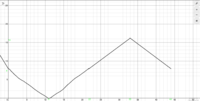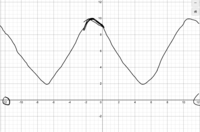# Need help finishing two functions of a graph

##### New memberThese are the two graphs in question. I know the first graph has a midline of 8 and an amplitude of 8 but the period and the phase shift are what is really difficult for me.
As for the second graph is a bit harder since it doesn't have many definitive points on the graph. Even the midline and amplitude are bugging me since its y != 9 when x = 0. the endpoints are -12 and 12 with the peak of the graph being x=10 and min being x = 2.

#### Dr.Peterson

##### Elite Member
Are these meant to be piecewise linear functions, or (very) rough sketches of sines? I'll assume the latter.

To find the period, look for the distance between equivalent points of two cycles. On the first, you just have one cycle, which starts (on the midline) at x=0 and ends (on the midline again) at 44, as marked. So the period is 44 - 0 = 44. There is no phase shift.

The second has a phase shift. Don't look at the y-intercept; that doesn't help with period and phase shift, as it depends on details of the function itself. The midline is the average of the high and low values, 10 and 2. What is that? To find the period, you need a better picture; it is not clear exactly where it crosses the midline, or even where the peaks are. Please show us the actual problem you are working on, so we can see what's given. Based only on what you show, I'd find the distance from peak to peak rather than midline to midline, and use my best guess (-7 to 5, or -1 to 11).